# Thermal Analysis of Disc Brake

This example analyses the temperature distribution of a disc brake. Disc brakes absorb the translational mechanical energy through friction and transform it into the thermal energy, which then dissipates. The transient thermal analysis of a disc brake is critical because the friction and braking performance decreases at high temperatures. Therefore, disc brakes must not exceed a given temperature limit during operation.

This example simulates the disc behavior in two steps:

• Perform a highly detailed simulation of the brake pad moving around the disc. Because the computational cost is high, this part of the example only simulates one half revolution (25 ms).

• Simulate full braking when the car goes from 100 km/h to 0 km/h in 2.75 seconds, and then remains stopped for 2.25 more seconds in order to allow the heat in the disc to dissipate.

The example uses a vehicle model in Simscape Driveline™ to obtain the time dependency of the dissipated power.

### Point Heat Source Moving Around the Disc

Simulate a circular brake pad moving around the disc. This detailed simulation over a short timescale models the heat source as a point moving around the disc.

First, create a thermal transient model.

`model = createpde('thermal','transient');`

Import the disc geometry.

`importGeometry(model,'brake_disc.stl');`

Plot the geometry with the face labels.

```figure pdegplot(model,'FaceLabels','on'); view([-5 -47])```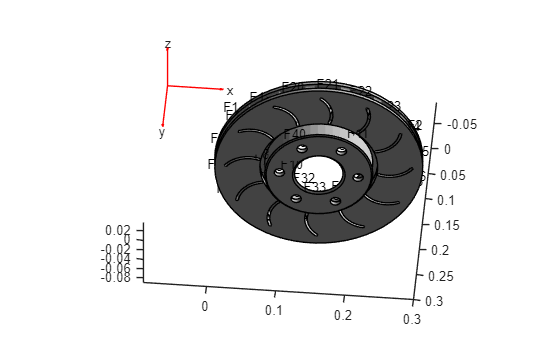Generate a fine mesh with a small target maximum element edge length. The resulting mesh has more than 130000 nodes (degrees of freedom).

`generateMesh(model,'Hmax',0.005);`

Plot the mesh.

```figure pdemesh(model) view([0,90])```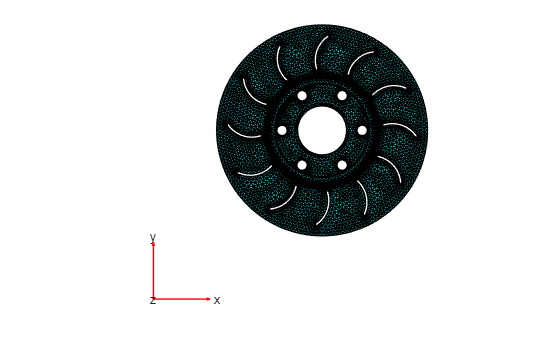Specify the thermal properties of the material.

```thermalProperties(model,'ThermalConductivity',100, ... 'MassDensity',8000, ... 'SpecificHeat',500);```

Specify the boundary conditions. All the faces are exposed to air, so there will be free convection.

```thermalBC(model,'Face',1:model.Geometry.NumFaces, ... 'ConvectionCoefficient',10, ... 'AmbientTemperature',30);```

Model the moving heat source by using a function handle that defines the thermal load as a function of space and time. For the definition of the `movingHeatSource` function, see the Heat Source Functions section at the bottom of this page.

```thermalBC(model,'Face',11,'HeatFlux',@movingHeatSource); thermalBC(model,'Face',4,'HeatFlux',@movingHeatSource); ```

Specify the initial temperature.

`thermalIC(model,30);`

Solve the model for the time steps from 0 to 25 ms.

```tlist = linspace(0,0.025,100); % Half revolution R1 = solve(model,tlist);```

Plot the temperature distribution at 25 ms.

```figure('units','normalized','outerposition',[0 0 1 1]) pdeplot3D(model,'ColorMapData',R1.Temperature(:,end))```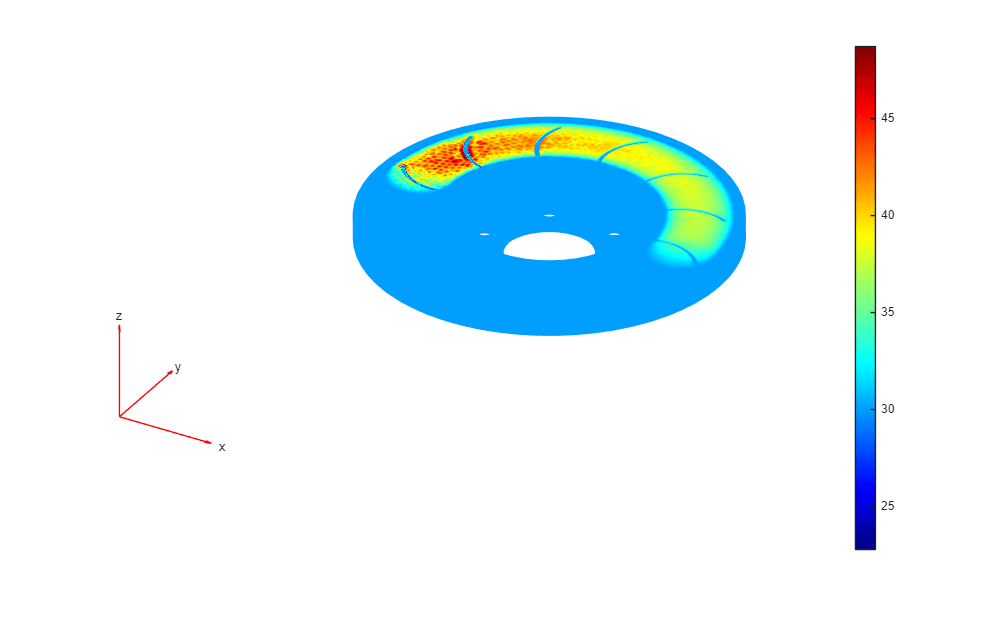The `animation` function visualizes the solution for all time steps. To play the animation, use this command:

`animation(model,R1)`

Because the heat diffusion time is much longer than the period of a revolution, you can simplify the heat source for the long simulation.

### Static Ring Heat Source

Now find the disc temperatures for a longer period of time. Because the heat does not have time to diffuse during a revolution, it is reasonable to approximate the heat source with a static heat source in the shape of the path of the brake pad.

Compute the heat flow applied to the disc as a function of time. To do this, use a Simscape Driveline™ model of a four-wheeled, 2000 kg vehicle that brakes from 100 km/h to 0 km/h in approximately 2.75 s.

```driveline_model = 'DrivelineVehicle_isothermal'; open_system(driveline_model);```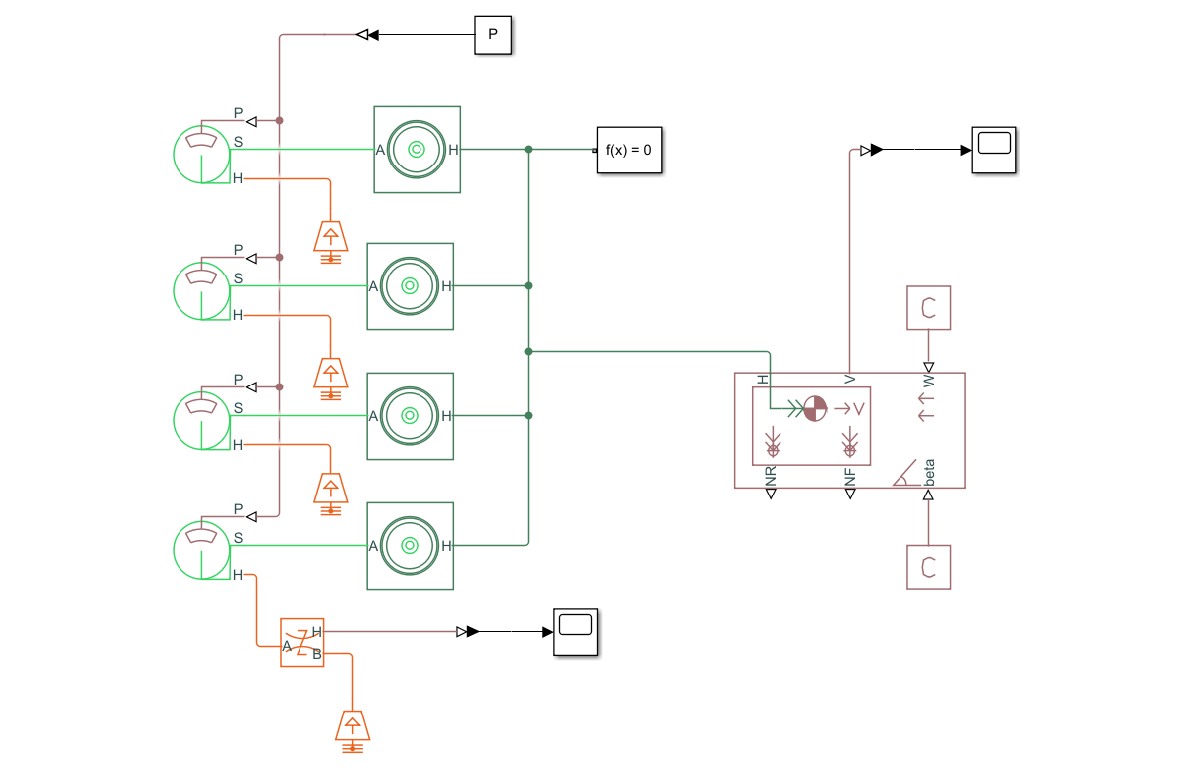```M = 2000; % kg V0 = 27.8; % m/s, which is around 100 km/h P = 277; % bar simOut = sim(driveline_model); heatFlow = simOut.simlog.Heat_Flow_Rate_Sensor.Q.series.values; tout = simOut.tout;```

Obtain the time-dependent heat flow by using the results from the Simscape Driveline model.

```drvln = struct(); drvln.tout = tout; drvln.heatFlow = heatFlow;```

Generate a mesh.

`generateMesh(model);`

Specify the boundary condition as a function handle. For the definition of the `ringHeatSource` function, see the Heat Source Functions section at the bottom of this page.

```thermalBC(model,'Face',11, ... 'HeatFlux',@(r,s)ringHeatSource(r,s,drvln)); thermalBC(model,'Face',4, ... 'HeatFlux',@(r,s)ringHeatSource(r,s,drvln)); ```

Solve the model for times from 0 to 5 seconds.

```tlist = linspace(0,5,250); R2 = solve(model,tlist);```

Plot the temperature distribution at the final time step t = 5 seconds.

```figure('units','normalized','outerposition',[0 0 1 1]) pdeplot3D(model,'ColorMapData',R2.Temperature(:,end))```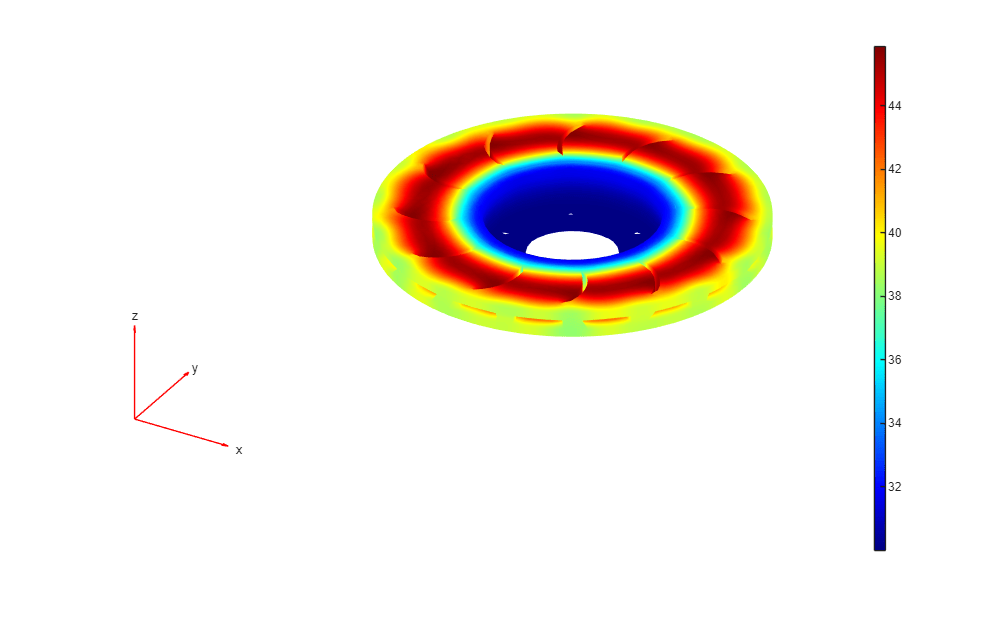The `animation` function visualizes the solution for all time steps. To play the animation, use the following command:

`animation(model,R2)`

Find the maximum temperature of the disc. The maximum temperature is low enough to ensure that the braking pad performs as expected.

`Tmax = max(max(R2.Temperature))`
```Tmax = 52.2895 ```

### Heat Source Functions for Moving and Static Heat Sources

```function F = movingHeatSource(region,state) % Parameters --------- R = 0.115; % Distance from center of disc to center of braking pad r = 0.025; % Radius of braking pad xc = 0.15; % x-coordinate of center of disc yc = 0.15; % y-coordinate of center of disc T = 0.05; % Period of 1 revolution of disc power = 35000; % Braking power in watts Tambient = 30; % Ambient temperature (for convection) h = 10; % Convection heat transfer coefficient in W/m^2*K %-------------------- theta = 2*pi/T*state.time; xs = xc + R*cos(theta); ys = yc + R*sin(theta); x = region.x; y = region.y; F = h*(Tambient - state.u); % Convection if isnan(state.time) F = nan(1,numel(x)); end idx = (x - xs).^2 + (y - ys).^2 <= r^2; F(1,idx) = 0.5*power/(pi*r.^2); % 0.5 because there are 2 faces end```
```function F = ringHeatSource(region,state,driveline) % Parameters --------- R = 0.115; % Distance from center of disc to center of braking pad r = 0.025; % Radius of braking pad xc = 0.15; % x-coordinate of center of disc yc = 0.15; % y-coordinate of center of disc % Braking power in watts power = interp1(driveline.tout,driveline.heatFlow,state.time); Tambient = 30; % Ambient temperature (for convection) h = 10; % Convection heat transfer coefficient in W/m^2*K Tf = 2.5; % Time in seconds %-------------------- x = region.x; y = region.y; F = h*(Tambient - state.u); % Convection if isnan(state.time) F = nan(1,numel(x)); end if state.time < Tf rad = sqrt((x - xc).^2 + (y - yc).^2); idx = rad >= R-r & rad <= R+r; area = pi*( (R+r)^2 - (R-r)^2 ); F(1,idx) = 0.5*power/area; % 0.5 because there are 2 faces end end```

## Support평가판 신청